# Using =VLOOKUP() in Excel when the lookup_value can be in column 1 or column 2 of the table_arrayon
Hello,

Suppose you have a spreadsheet which contains a 3-column table.

What formula would enable a user to enter a value from either the 1st or 2nd column but always return the values found in the 2nd and 3rd columns of the corresponding row?

For example, the table in the following screenshots contains three ways (written in columns 1 & 2 and numeric in column 3) to designate months. Also included is an input box (yellow) in which a user can enter a given month — but only in one of the written forms from the 1st (Fig. 1) or 2nd (Fig. 2) columns of the table (ie numeric entries are not allowed):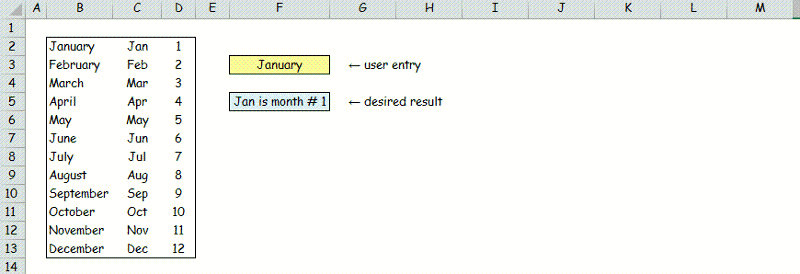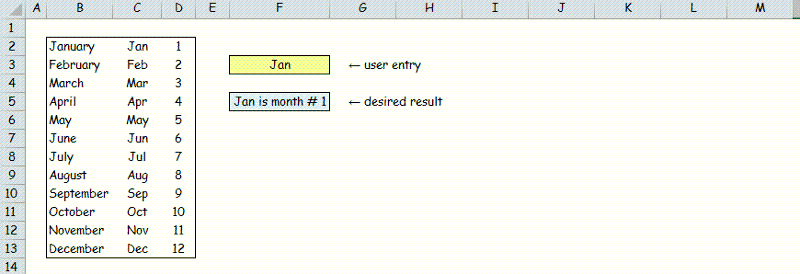What formula would return the result shown in the blue box regardless of which of the two written forms was utilized by the user?

Notes:
1) the use of months in this example is arbitrary (ie please avoid solutions which include date or time functions).
2) Please disregard the fact that each of the 2nd-column values in the table not only contains exactly three letters but the first three letters of the corresponding 1st-column values. That is just a coincidence of this example but does not apply where I plan to use the solution.

The best approach I've found so far involves naming two defined ranges:

• "Months3" which includes all three columns (Fig. 3) and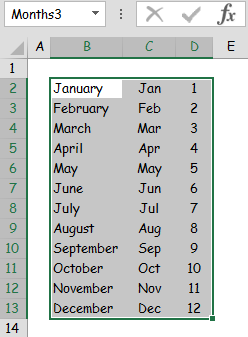• "Months2" which includes the 2nd & 3rd columns but not the 1st column (Fig. 4):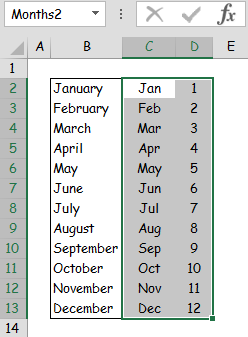With the named ranges and utilizing the =VLOOKUP() function, two separate formulas can be written, one for inputs from the 1st column (Fig. 5):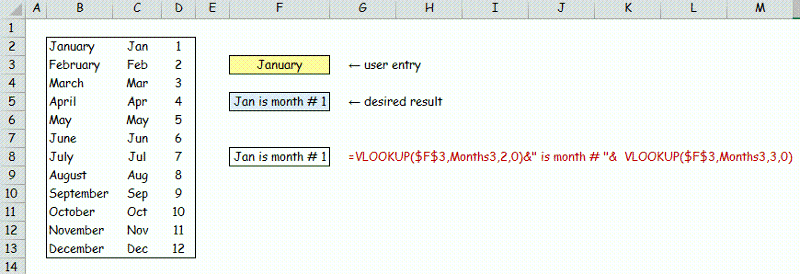and one for inputs from the 2nd column (Fig. 6):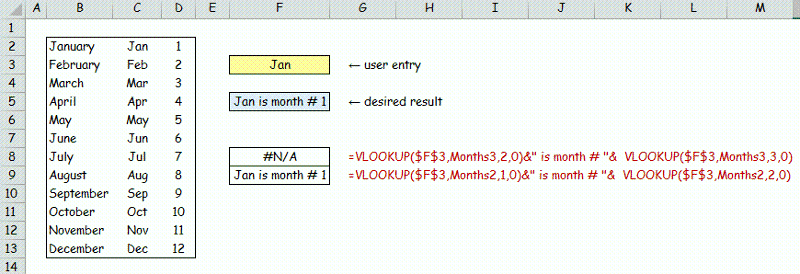Note: the function =GET.CELL(6,F8) enables the display (red font) of the adjacent formulas in F8 & F9.

I believe this approach is usable but as can be seen in Fig. 6, each formula works for entries from one of the first two columns but returns #N/A for the other. Therefore, if someone can show me how to nest the two =VLOOKUP() formulas into one so that the 1st column is searched first and the 2nd column is searched only if the 1st column search fails, I think that will do it.

Alternatively, I am interested in learning different but simpler ways to arrive at the same endpoint.

Thanks
Comment
Watch Question

Do more withEXPERT OFFICE® is a registered trademark of EXPERTS EXCHANGE®
I would use something like:
``````If(iserr(vlookup(f3,\$range,3,False)),vlookup(f3,\$range2,2,False),vlookup(f3,\$range,3,False)
``````

Basically, check to see if looking up f3 in column 1 of \$range (3 columns) returns a value - if so, ISERR returns false, and the 2d formula (which is the same as the test formula) is used to get a result from the 3rd column. If the test lookup fails and returns an error (might return null, I'll do some research), then use the 2d formula, which looks up the value in column 1 of the 2-column range and returns the value in column 2 thereof.

Commented:
Oops, forgot to attach the file. Here it is:

c-EE-2014-11-18.xlsm
Well - I went and did a test, and found this option:
``````=IFNA(VLOOKUP(F1,range3col,3,FALSE),VLOOKUP(F1,range2col,2,FALSE))
``````
It's basically the same logic, but there's 2 changes:
1) If the lookup fails, it return #N/A, which is NOT an error value;
2)There's an IFNA function (not sure when that was added) that makes the code easier to read

In the formula above, F1 is the input cell and range3col and range2col refer to named ranges

By the way, the FALSE argument just tell VLOOKUP not to try to an interpolated match - if it's looking for "b" in the list "a","c","e" it will return "a" if the 4th argument is null or True.
By the way, I would never post a .xlsm file on a public board - anyone who loads it could get zapped by the code - better to post either an .xlsx (no code) file or, if you're working on VBA code,  just post the module code as text files (.bas or .txt).
Top Expert 2010

Commented:
I'm kind of partial to this construction, as it does not rely on letting VLOOKUP fail:

=LEFT(F3,3)&" is month #"&INDEX(D2:D13,MATCH(F3,IF(LEN(F3)=3,C2:C13,B2:B13),0))
You could use wildcard in VLOOKUP. So

=F3&" is month #"&VLOOKUP(F3&"*",B2:D13,3,0)

would work.

Kris
BTW, replace first F3 with LEFT(F3,3)

Kris

Commented:
Thanks for all the responses. I'm working through the formulas they contain and will respond — but this one doesn't need any "working through" :
eflamm >>>
…I would never post a .xlsm file on a public board - anyone who loads it could get zapped by the code…
Thanks a bunch for the heads-up. I was not aware of that risk until reading your post. I would feel terrible if something I uploaded caused problems for someone so I will be careful to not do it again.
@krishnakrkc - OP specifically said that that the fact that the 2 columns were subsets was not part of the specification - so the first column could be Apple and the second could be Broccoli. I don't see how a wildcard constructor would address those inputs.

Commented:
Please note the following from my original question:
Please disregard the fact that each of the 2nd-column values in the table not only contains exactly three letters but the first three letters of the corresponding 1st-column values. That is just a coincidence of this example but does not apply where I plan to use the solution.
Most Valuable Expert 2011
Top Expert 2011
Commented:
Perhaps:

=INDEX(C2:C13&" is month # "&D2:D13,IFERROR(MATCH(F3,B2:B13,0),MATCH(F3,C2:C13,0)))
Finance Analyst

Commented:
@Eric Flamm - #NA is an error value. The IFNA function came in Excel 2007 along with IFERROR which covers all Error values; #NA, #Ref, #Div0 etc etc.

Thanks
Rob H

Commented:
Eric Flamm >>
I would use something like:
If(iserr(vlookup(f3,\$range,3,False)),vlookup(f3,\$range2,2,False),vlookup(f3,\$range,3,False)
Thanks for the feedback Eric but I cannot get this formula, even when completed, to return a meaningful result.

Eric Flamm >>
Well - I went and did a test, and found this option:
=IFNA(VLOOKUP(F1,range3col,3,FALSE),VLOOKUP(F1,range2col,2,FALSE))
This formula returns a value from the 3rd column but the objective is to return values from both the 2nd & 3rd columns.

Commented:
Rory Archibald >>
Perhaps:
=INDEX(C2:C13&" is month # "&D2:D13,IFERROR(MATCH(F3,B2:B13,0),MATCH(F3,C2:C13,0)))
Thanks Rory. That is exactly what I was looking for.

Commented:
Thanks

Do more withSubmit tech questions to Ask the Experts™ at any time to receive solutions, advice, and new ideas from leading industry professionals.